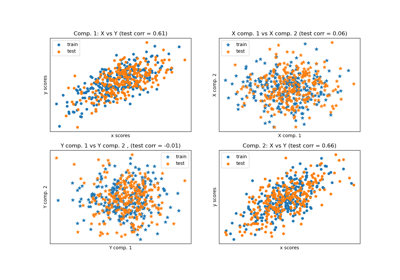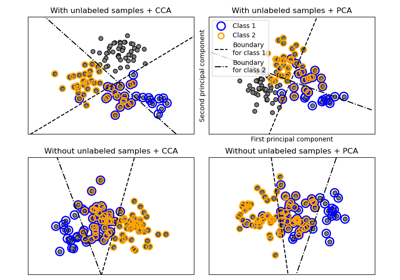# sklearn.cross_decomposition.CCA¶

class sklearn.cross_decomposition.CCA(n_components=2, *, scale=True, max_iter=500, tol=1e-06, copy=True)[source]

Canonical Correlation Analysis, also known as “Mode B” PLS.

Read more in the User Guide.

Parameters:
n_componentsint, default=2

Number of components to keep. Should be in [1, min(n_samples, n_features, n_targets)].

scalebool, default=True

Whether to scale X and Y.

max_iterint, default=500

The maximum number of iterations of the power method.

tolfloat, default=1e-06

The tolerance used as convergence criteria in the power method: the algorithm stops whenever the squared norm of u_i - u_{i-1} is less than tol, where u corresponds to the left singular vector.

copybool, default=True

Whether to copy X and Y in fit before applying centering, and potentially scaling. If False, these operations will be done inplace, modifying both arrays.

Attributes:
x_weights_ndarray of shape (n_features, n_components)

The left singular vectors of the cross-covariance matrices of each iteration.

y_weights_ndarray of shape (n_targets, n_components)

The right singular vectors of the cross-covariance matrices of each iteration.

The loadings of X.

The loadings of Y.

x_rotations_ndarray of shape (n_features, n_components)

The projection matrix used to transform X.

y_rotations_ndarray of shape (n_features, n_components)

The projection matrix used to transform Y.

coef_ndarray of shape (n_targets, n_features)

The coefficients of the linear model such that Y is approximated as Y = X @ coef_.T + intercept_.

intercept_ndarray of shape (n_targets,)

The intercepts of the linear model such that Y is approximated as Y = X @ coef_.T + intercept_.

New in version 1.1.

n_iter_list of shape (n_components,)

Number of iterations of the power method, for each component.

n_features_in_int

Number of features seen during fit.

feature_names_in_ndarray of shape (n_features_in_,)

Names of features seen during fit. Defined only when X has feature names that are all strings.

New in version 1.0.

PLSCanonical

Partial Least Squares transformer and regressor.

PLSSVD

Partial Least Square SVD.

Examples

>>> from sklearn.cross_decomposition import CCA
>>> X = [[0., 0., 1.], [1.,0.,0.], [2.,2.,2.], [3.,5.,4.]]
>>> Y = [[0.1, -0.2], [0.9, 1.1], [6.2, 5.9], [11.9, 12.3]]
>>> cca = CCA(n_components=1)
>>> cca.fit(X, Y)
CCA(n_components=1)
>>> X_c, Y_c = cca.transform(X, Y)


Methods

 fit(X, Y) Fit model to data. fit_transform(X[, y]) Learn and apply the dimension reduction on the train data. get_feature_names_out([input_features]) Get output feature names for transformation. Get metadata routing of this object. get_params([deep]) Get parameters for this estimator. inverse_transform(X[, Y]) Transform data back to its original space. predict(X[, copy]) Predict targets of given samples. score(X, y[, sample_weight]) Return the coefficient of determination of the prediction. set_output(*[, transform]) Set output container. set_params(**params) Set the parameters of this estimator. set_predict_request(*[, copy]) Request metadata passed to the predict method. set_score_request(*[, sample_weight]) Request metadata passed to the score method. set_transform_request(*[, copy]) Request metadata passed to the transform method. transform(X[, Y, copy]) Apply the dimension reduction.
fit(X, Y)[source]

Fit model to data.

Parameters:
Xarray-like of shape (n_samples, n_features)

Training vectors, where n_samples is the number of samples and n_features is the number of predictors.

Yarray-like of shape (n_samples,) or (n_samples, n_targets)

Target vectors, where n_samples is the number of samples and n_targets is the number of response variables.

Returns:
selfobject

Fitted model.

fit_transform(X, y=None)[source]

Learn and apply the dimension reduction on the train data.

Parameters:
Xarray-like of shape (n_samples, n_features)

Training vectors, where n_samples is the number of samples and n_features is the number of predictors.

yarray-like of shape (n_samples, n_targets), default=None

Target vectors, where n_samples is the number of samples and n_targets is the number of response variables.

Returns:
selfndarray of shape (n_samples, n_components)

Return x_scores if Y is not given, (x_scores, y_scores) otherwise.

get_feature_names_out(input_features=None)[source]

Get output feature names for transformation.

The feature names out will prefixed by the lowercased class name. For example, if the transformer outputs 3 features, then the feature names out are: ["class_name0", "class_name1", "class_name2"].

Parameters:
input_featuresarray-like of str or None, default=None

Only used to validate feature names with the names seen in fit.

Returns:
feature_names_outndarray of str objects

Transformed feature names.

Get metadata routing of this object.

Please check User Guide on how the routing mechanism works.

Returns:

A MetadataRequest encapsulating routing information.

get_params(deep=True)[source]

Get parameters for this estimator.

Parameters:
deepbool, default=True

If True, will return the parameters for this estimator and contained subobjects that are estimators.

Returns:
paramsdict

Parameter names mapped to their values.

inverse_transform(X, Y=None)[source]

Transform data back to its original space.

Parameters:
Xarray-like of shape (n_samples, n_components)

New data, where n_samples is the number of samples and n_components is the number of pls components.

Yarray-like of shape (n_samples, n_components)

New target, where n_samples is the number of samples and n_components is the number of pls components.

Returns:
X_reconstructedndarray of shape (n_samples, n_features)

Return the reconstructed X data.

Y_reconstructedndarray of shape (n_samples, n_targets)

Return the reconstructed X target. Only returned when Y is given.

Notes

This transformation will only be exact if n_components=n_features.

predict(X, copy=True)[source]

Predict targets of given samples.

Parameters:
Xarray-like of shape (n_samples, n_features)

Samples.

copybool, default=True

Whether to copy X and Y, or perform in-place normalization.

Returns:
y_predndarray of shape (n_samples,) or (n_samples, n_targets)

Returns predicted values.

Notes

This call requires the estimation of a matrix of shape (n_features, n_targets), which may be an issue in high dimensional space.

score(X, y, sample_weight=None)[source]

Return the coefficient of determination of the prediction.

The coefficient of determination $$R^2$$ is defined as $$(1 - \frac{u}{v})$$, where $$u$$ is the residual sum of squares ((y_true - y_pred)** 2).sum() and $$v$$ is the total sum of squares ((y_true - y_true.mean()) ** 2).sum(). The best possible score is 1.0 and it can be negative (because the model can be arbitrarily worse). A constant model that always predicts the expected value of y, disregarding the input features, would get a $$R^2$$ score of 0.0.

Parameters:
Xarray-like of shape (n_samples, n_features)

Test samples. For some estimators this may be a precomputed kernel matrix or a list of generic objects instead with shape (n_samples, n_samples_fitted), where n_samples_fitted is the number of samples used in the fitting for the estimator.

yarray-like of shape (n_samples,) or (n_samples, n_outputs)

True values for X.

sample_weightarray-like of shape (n_samples,), default=None

Sample weights.

Returns:
scorefloat

$$R^2$$ of self.predict(X) w.r.t. y.

Notes

The $$R^2$$ score used when calling score on a regressor uses multioutput='uniform_average' from version 0.23 to keep consistent with default value of r2_score. This influences the score method of all the multioutput regressors (except for MultiOutputRegressor).

set_output(*, transform=None)[source]

Set output container.

See Introducing the set_output API for an example on how to use the API.

Parameters:
transform{“default”, “pandas”}, default=None

Configure output of transform and fit_transform.

• "default": Default output format of a transformer

• "pandas": DataFrame output

• None: Transform configuration is unchanged

Returns:
selfestimator instance

Estimator instance.

set_params(**params)[source]

Set the parameters of this estimator.

The method works on simple estimators as well as on nested objects (such as Pipeline). The latter have parameters of the form <component>__<parameter> so that it’s possible to update each component of a nested object.

Parameters:
**paramsdict

Estimator parameters.

Returns:
selfestimator instance

Estimator instance.

set_predict_request(*, copy: Union[bool, None, str] = '$UNCHANGED$') CCA[source]

Request metadata passed to the predict method.

Note that this method is only relevant if enable_metadata_routing=True (see sklearn.set_config). Please see User Guide on how the routing mechanism works.

The options for each parameter are:

• True: metadata is requested, and passed to predict if provided. The request is ignored if metadata is not provided.

• False: metadata is not requested and the meta-estimator will not pass it to predict.

• None: metadata is not requested, and the meta-estimator will raise an error if the user provides it.

• str: metadata should be passed to the meta-estimator with this given alias instead of the original name.

The default (sklearn.utils.metadata_routing.UNCHANGED) retains the existing request. This allows you to change the request for some parameters and not others.

New in version 1.3.

Note

This method is only relevant if this estimator is used as a sub-estimator of a meta-estimator, e.g. used inside a pipeline.Pipeline. Otherwise it has no effect.

Parameters:
copystr, True, False, or None, default=sklearn.utils.metadata_routing.UNCHANGED

Metadata routing for copy parameter in predict.

Returns:
selfobject

The updated object.

set_score_request(*, sample_weight: Union[bool, None, str] = '$UNCHANGED$') CCA[source]

Request metadata passed to the score method.

Note that this method is only relevant if enable_metadata_routing=True (see sklearn.set_config). Please see User Guide on how the routing mechanism works.

The options for each parameter are:

• True: metadata is requested, and passed to score if provided. The request is ignored if metadata is not provided.

• False: metadata is not requested and the meta-estimator will not pass it to score.

• None: metadata is not requested, and the meta-estimator will raise an error if the user provides it.

• str: metadata should be passed to the meta-estimator with this given alias instead of the original name.

The default (sklearn.utils.metadata_routing.UNCHANGED) retains the existing request. This allows you to change the request for some parameters and not others.

New in version 1.3.

Note

This method is only relevant if this estimator is used as a sub-estimator of a meta-estimator, e.g. used inside a pipeline.Pipeline. Otherwise it has no effect.

Parameters:
sample_weightstr, True, False, or None, default=sklearn.utils.metadata_routing.UNCHANGED

Metadata routing for sample_weight parameter in score.

Returns:
selfobject

The updated object.

set_transform_request(*, copy: Union[bool, None, str] = '$UNCHANGED$') CCA[source]

Request metadata passed to the transform method.

Note that this method is only relevant if enable_metadata_routing=True (see sklearn.set_config). Please see User Guide on how the routing mechanism works.

The options for each parameter are:

• True: metadata is requested, and passed to transform if provided. The request is ignored if metadata is not provided.

• False: metadata is not requested and the meta-estimator will not pass it to transform.

• None: metadata is not requested, and the meta-estimator will raise an error if the user provides it.

• str: metadata should be passed to the meta-estimator with this given alias instead of the original name.

The default (sklearn.utils.metadata_routing.UNCHANGED) retains the existing request. This allows you to change the request for some parameters and not others.

New in version 1.3.

Note

This method is only relevant if this estimator is used as a sub-estimator of a meta-estimator, e.g. used inside a pipeline.Pipeline. Otherwise it has no effect.

Parameters:
copystr, True, False, or None, default=sklearn.utils.metadata_routing.UNCHANGED

Metadata routing for copy parameter in transform.

Returns:
selfobject

The updated object.

transform(X, Y=None, copy=True)[source]

Apply the dimension reduction.

Parameters:
Xarray-like of shape (n_samples, n_features)

Samples to transform.

Yarray-like of shape (n_samples, n_targets), default=None

Target vectors.

copybool, default=True

Whether to copy X and Y, or perform in-place normalization.

Returns:
x_scores, y_scoresarray-like or tuple of array-like

Return x_scores if Y is not given, (x_scores, y_scores) otherwise.

## Examples using sklearn.cross_decomposition.CCA¶Compare cross decomposition methods

Compare cross decomposition methodsMultilabel classification

Multilabel classification